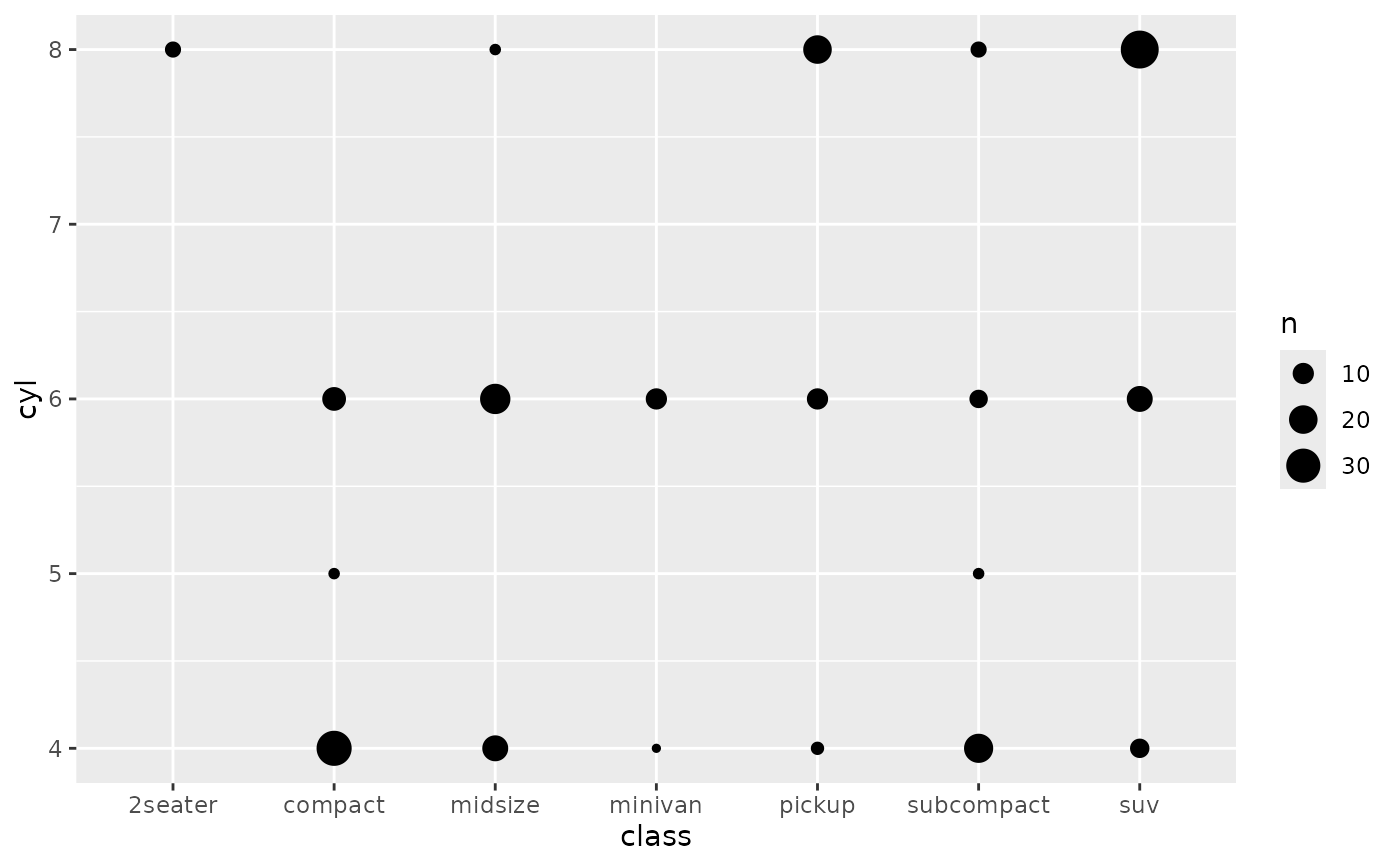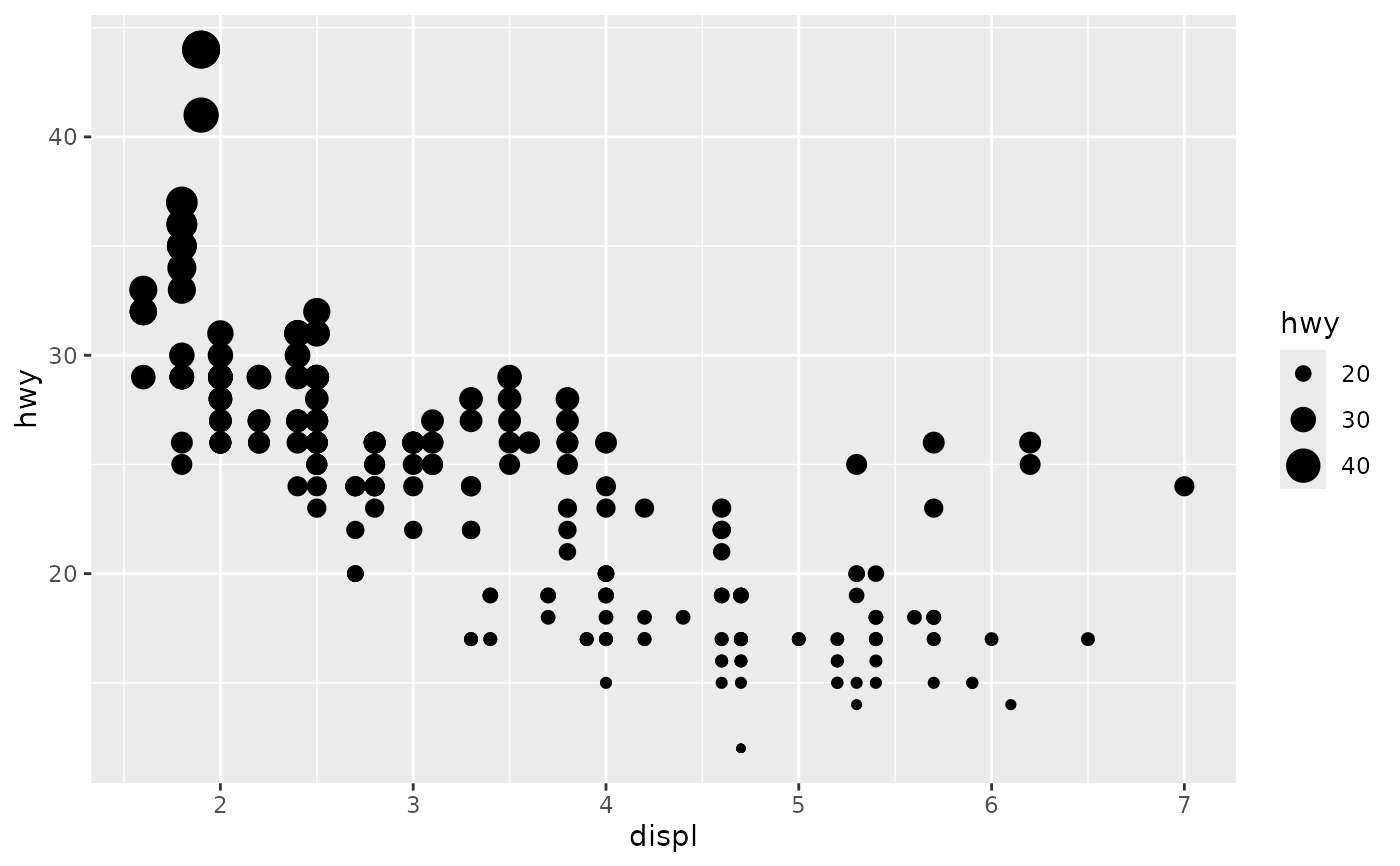scale_size() scales area, scale_radius() scales radius. The size aesthetic is most commonly used for points and text, and humans perceive the area of points (not their radius), so this provides for optimal perception. scale_size_area() ensures that a value of 0 is mapped to a size of 0. scale_size_binned() is a binned version of scale_size() that scales by area (but does not ensure 0 equals an area of zero). For a binned equivalent of scale_size_area() use scale_size_binned_area().

## Usage

scale_size(
name = waiver(),
breaks = waiver(),
labels = waiver(),
limits = NULL,
range = c(1, 6),
trans = "identity",
guide = "legend"
)

name = waiver(),
breaks = waiver(),
labels = waiver(),
limits = NULL,
range = c(1, 6),
trans = "identity",
guide = "legend"
)

scale_size_binned(
name = waiver(),
breaks = waiver(),
labels = waiver(),
limits = NULL,
range = c(1, 6),
n.breaks = NULL,
nice.breaks = TRUE,
trans = "identity",
guide = "bins"
)

scale_size_area(..., max_size = 6)

scale_size_binned_area(..., max_size = 6)

## Arguments

name

The name of the scale. Used as the axis or legend title. If waiver(), the default, the name of the scale is taken from the first mapping used for that aesthetic. If NULL, the legend title will be omitted.

breaks

One of:

• NULL for no breaks

• waiver() for the default breaks computed by the transformation object

• A numeric vector of positions

• A function that takes the limits as input and returns breaks as output (e.g., a function returned by scales::extended_breaks()). Also accepts rlang lambda function notation.

labels

One of:

• NULL for no labels

• waiver() for the default labels computed by the transformation object

• A character vector giving labels (must be same length as breaks)

• An expression vector (must be the same length as breaks). See ?plotmath for details.

• A function that takes the breaks as input and returns labels as output. Also accepts rlang lambda function notation.

limits

One of:

• NULL to use the default scale range

• A numeric vector of length two providing limits of the scale. Use NA to refer to the existing minimum or maximum

• A function that accepts the existing (automatic) limits and returns new limits. Also accepts rlang lambda function notation. Note that setting limits on positional scales will remove data outside of the limits. If the purpose is to zoom, use the limit argument in the coordinate system (see coord_cartesian()).

range

a numeric vector of length 2 that specifies the minimum and maximum size of the plotting symbol after transformation.

trans

For continuous scales, the name of a transformation object or the object itself. Built-in transformations include "asn", "atanh", "boxcox", "date", "exp", "hms", "identity", "log", "log10", "log1p", "log2", "logit", "modulus", "probability", "probit", "pseudo_log", "reciprocal", "reverse", "sqrt" and "time".

A transformation object bundles together a transform, its inverse, and methods for generating breaks and labels. Transformation objects are defined in the scales package, and are called <name>_trans (e.g., scales::boxcox_trans()). You can create your own transformation with scales::trans_new().

guide

A function used to create a guide or its name. See guides() for more information.

n.breaks

An integer guiding the number of major breaks. The algorithm may choose a slightly different number to ensure nice break labels. Will only have an effect if breaks = waiver(). Use NULL to use the default number of breaks given by the transformation.

nice.breaks

Logical. Should breaks be attempted placed at nice values instead of exactly evenly spaced between the limits. If TRUE (default) the scale will ask the transformation object to create breaks, and this may result in a different number of breaks than requested. Ignored if breaks are given explicitly.

...

Arguments passed on to continuous_scale

minor_breaks

One of:

• NULL for no minor breaks

• waiver() for the default breaks (one minor break between each major break)

• A numeric vector of positions

• A function that given the limits returns a vector of minor breaks. Also accepts rlang lambda function notation.

oob

One of:

• Function that handles limits outside of the scale limits (out of bounds). Also accepts rlang lambda function notation.

• The default (scales::censor()) replaces out of bounds values with NA.

• scales::squish() for squishing out of bounds values into range.

• scales::squish_infinite() for squishing infinite values into range.

na.value

Missing values will be replaced with this value.

expand

For position scales, a vector of range expansion constants used to add some padding around the data to ensure that they are placed some distance away from the axes. Use the convenience function expansion() to generate the values for the expand argument. The defaults are to expand the scale by 5% on each side for continuous variables, and by 0.6 units on each side for discrete variables.

position

For position scales, The position of the axis. left or right for y axes, top or bottom for x axes.

super

The super class to use for the constructed scale

max_size

Size of largest points.

## Note

Historically the size aesthetic was used for two different things: Scaling the size of object (like points and glyphs) and scaling the width of lines. From ggplot2 3.4.0 the latter has been moved to its own linewidth aesthetic. For backwards compatibility using size is still possible, but it is highly advised to switch to the new linewidth aesthetic for these cases.

scale_size_area() if you want 0 values to be mapped to points with size 0. scale_linewidth() if you want to scale the width of lines.

## Examples

p <- ggplot(mpg, aes(displ, hwy, size = hwy)) +
geom_point()
p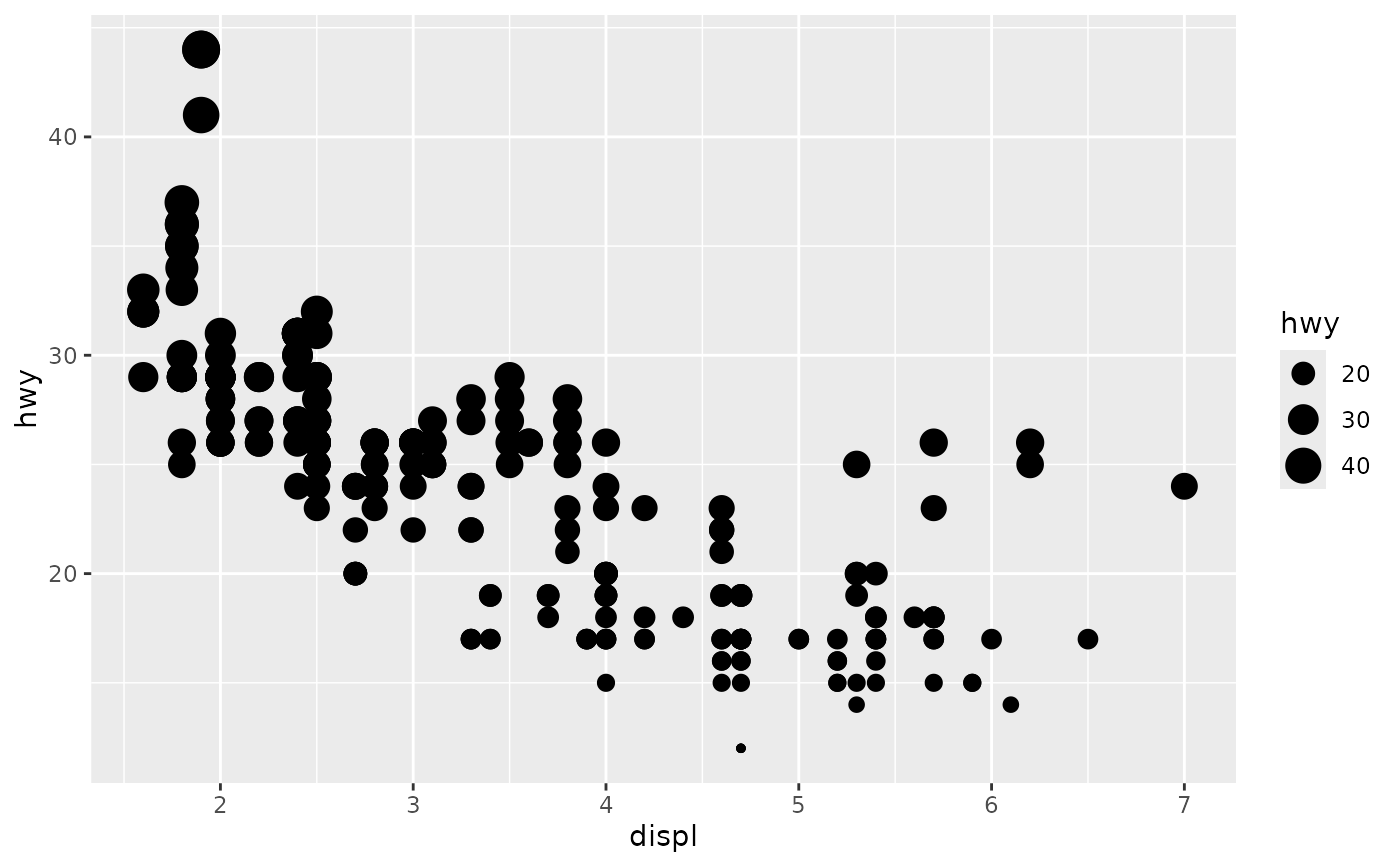p + scale_size("Highway mpg")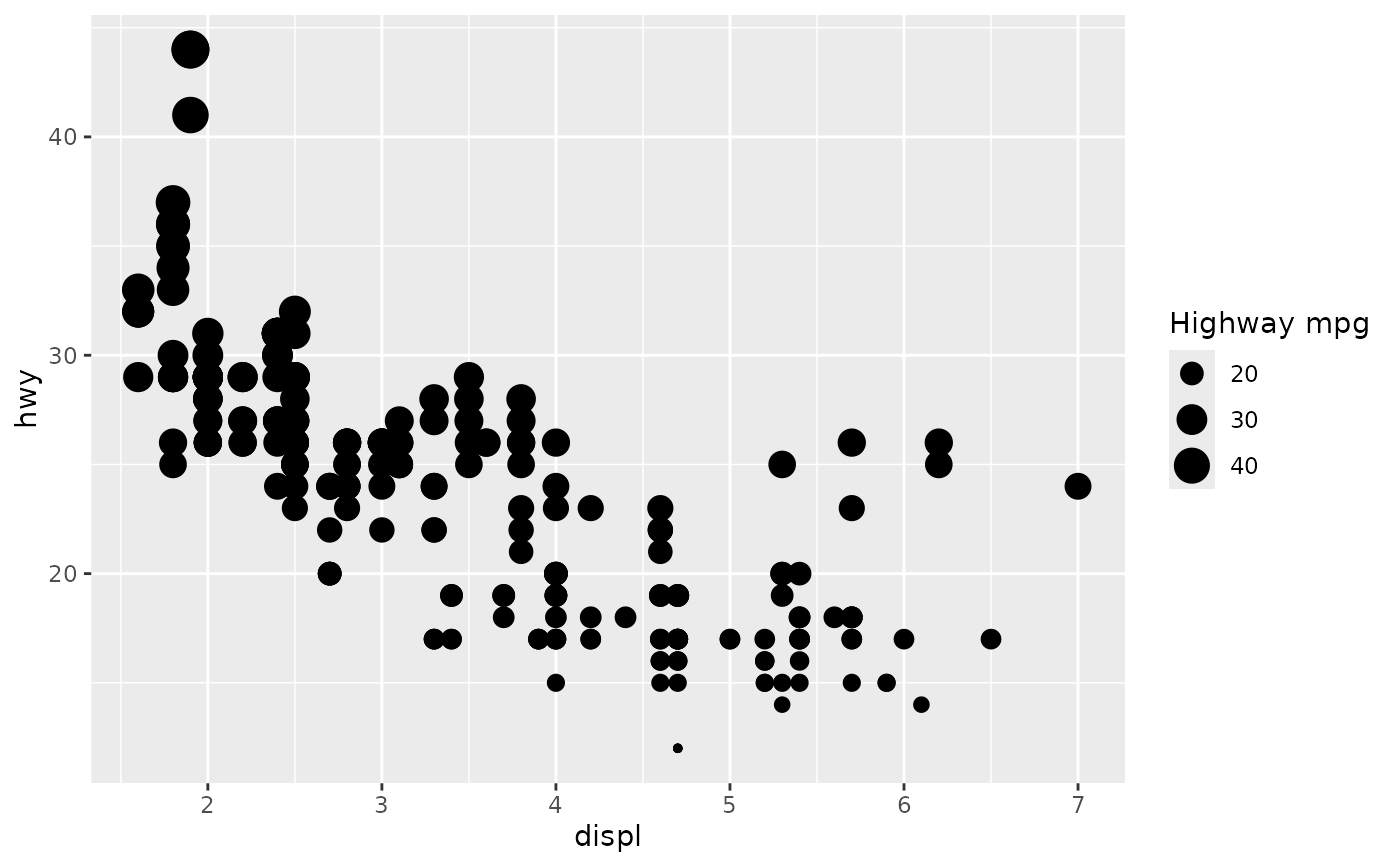p + scale_size(range = c(0, 10))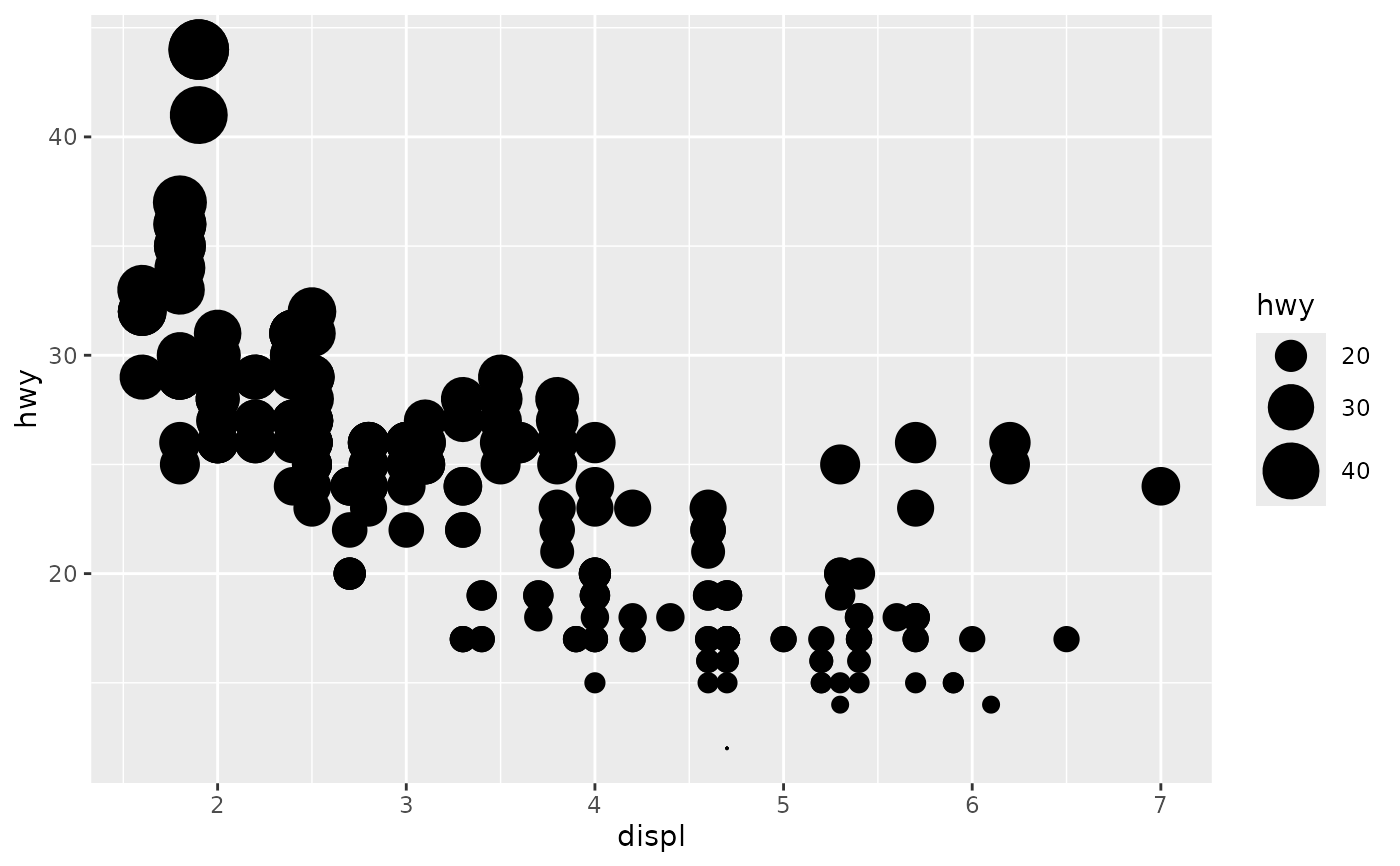# If you want zero value to have zero size, use scale_size_area:
p + scale_size_area()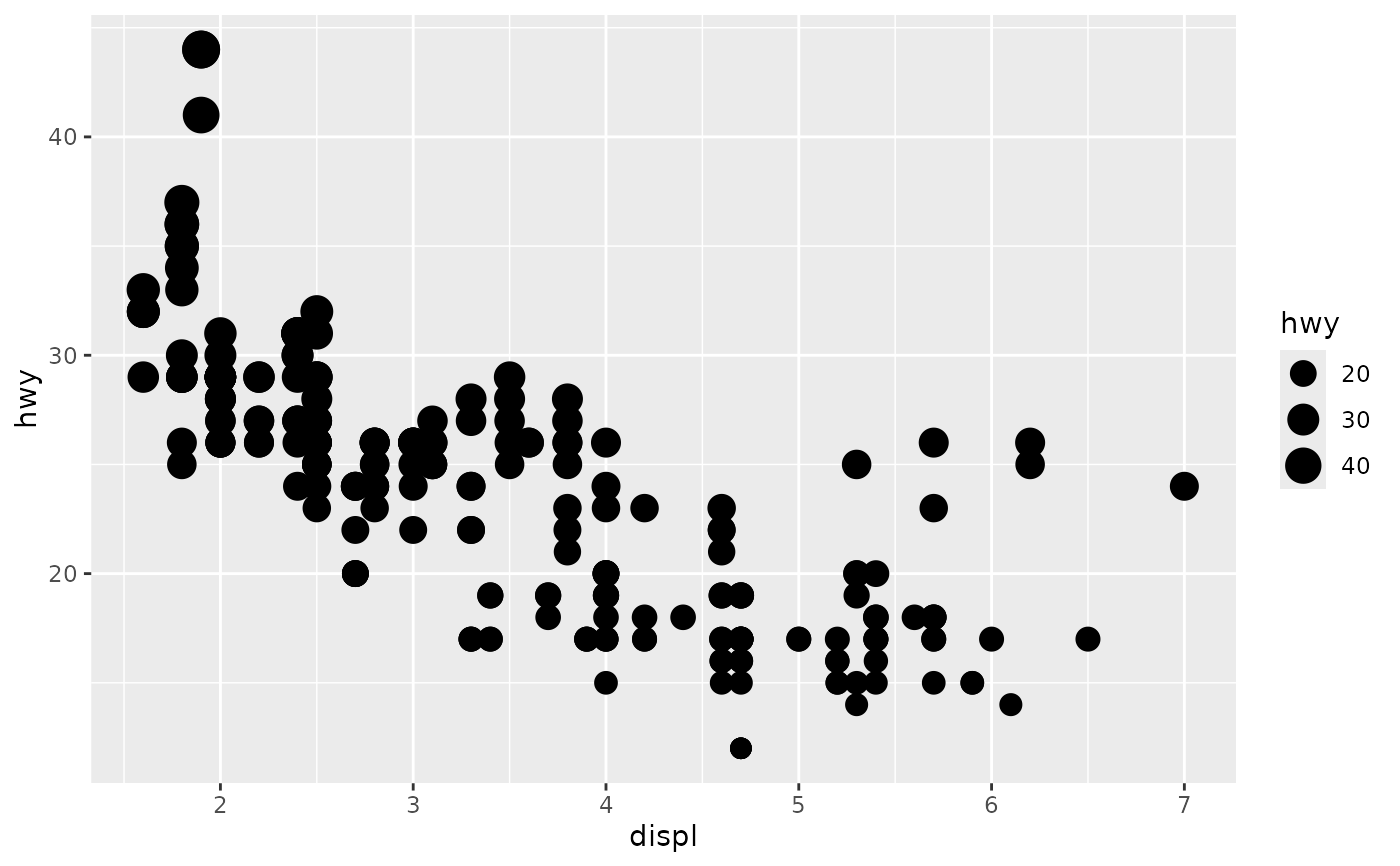# Binning can sometimes make it easier to match the scaled data to the legend
p + scale_size_binned()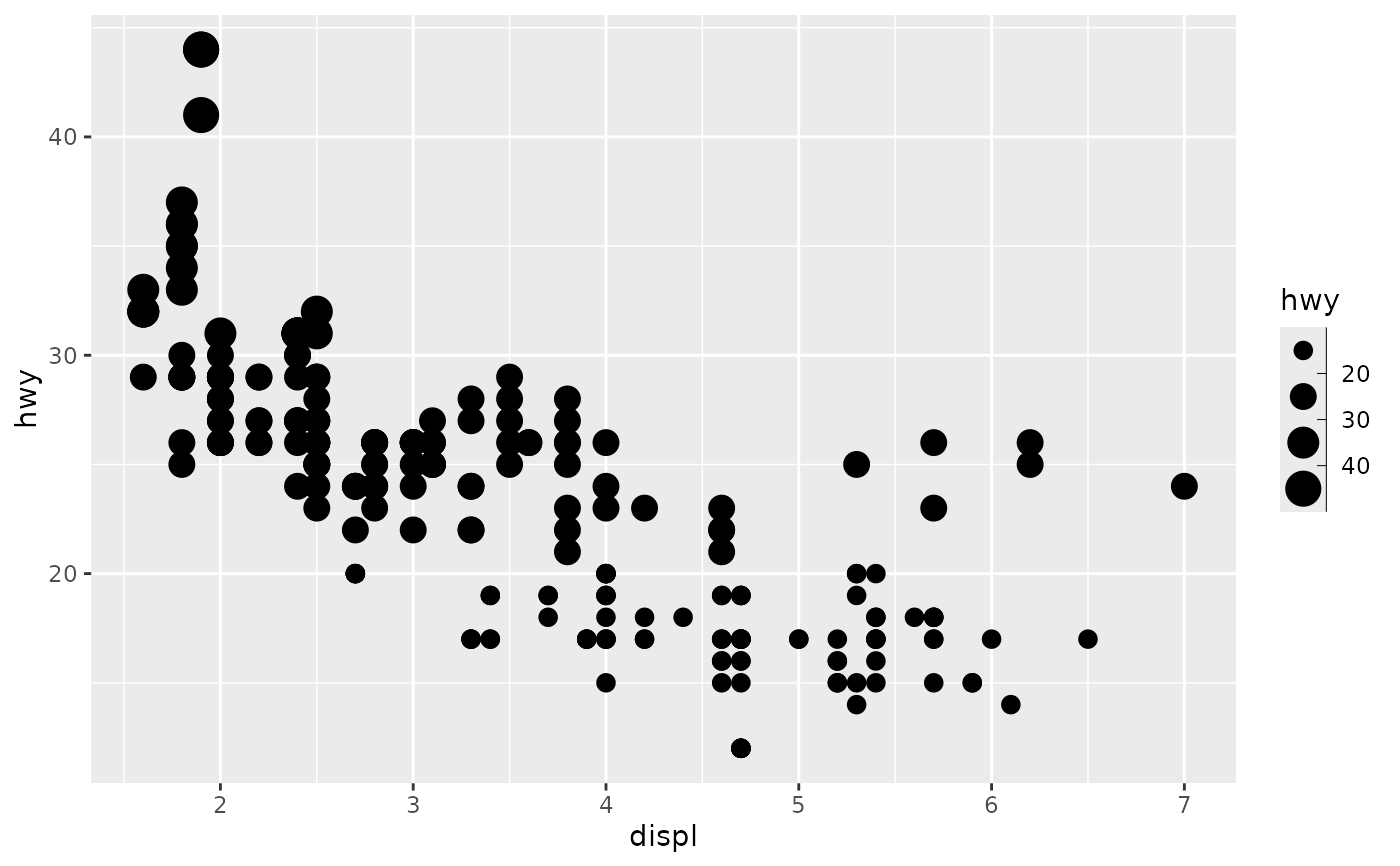# This is most useful when size is a count
ggplot(mpg, aes(class, cyl)) +
geom_count() +
scale_size_area()